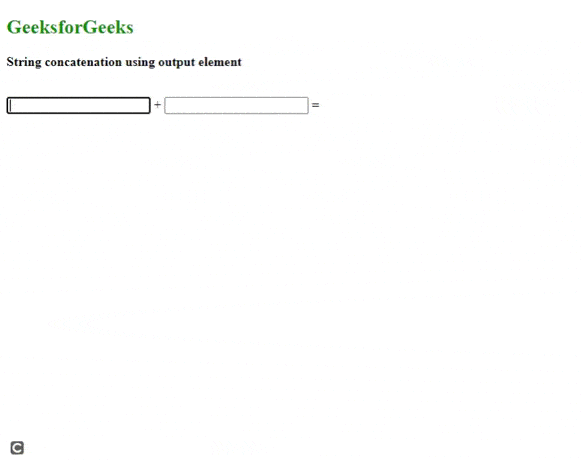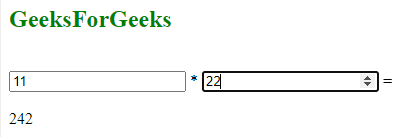Open In App

# How to define one or more forms the output element belongs to ?

The purpose of this article is basically to understand how we can define one or more forms in HTML and specify the output elements which belong to any particular form.

In simpler words, we are going to define a form with id as a reference to point out the output elements.

Approach: In order to define one or more forms, we simply use the <form> tag followed by the content of the form.  As it is in a container tag it terminates by </form> tag.

Now, for defining the output elements we use the <output> form attribute to specify the form it belongs to with the help of the form id.

The attributes used while specifying the output elements are:

• For
• Form
• Name

Syntax:

```<output name="variable"  for= "other variable"
form="form id"></output>```

Note: The “name” attribute is used to specify the output variable, “for” attribute is used to operate on the variable used within the form block and the “form” attribute is used to define the form through its id.

Example 1: The following code uses oninput attribute to perform the output during runtime.  The form id is “MyForm” and it concatenates two different texts by using form id as reference. The output element is specified within the form block.

## HTML

 ``` ` `<``html``>``  ``<``body``>``    ``<``h2` `style``=``"color: green"``>GeeksforGeeks`` ` `    ``<``p``><``b``>String concatenation using output element`` ` `    ``<``br` `/>``    ````    ``<``form` `id``=``"MyForm"` `          ``oninput``=``"second_output.value=val1.value+val2.value"``>`` ` `      ````      ``<``input` `type``=``"text"` `name``=``"val1"` `id``=``"val1"` `/> +``      ``<``input` `type``=``"text"` `name``=``"val2"` `id``=``"val2"` `/> =``      ````      ``<``output` `form``=``"MyForm"` `              ``name``=``"second_output"` `              ``for``=``"val1 val2"``>``      ````    ````  `````

Output:output element inside form

Example 2: In this example, we are going to define the output elements outside the form.

## HTML

 ```<``html``>``  ``<``body``>``    ``<``h2` `style``=``"color: green"``>GeeksForGeeks``    ``<``br` `/>``    ````    ``<``form` `id``=``"MyForm"` `          ``oninput``=``"first_output.value=a.value*b.value"``>`` ` `      ````      ``<``input` `type``=``"number"` `name``=``"a"` `id``=``"val1"` `/> *``      ``<``input` `type``=``"number"` `name``=``"b"` `id``=``"val2"` `/> =``    ````    ``<``br` `/>``    ````    ````    ``<``output` `form``=``"MyForm"` `            ``name``=``"first_output"` `            ``for``=``"val1 val2"``>``    ````  `````

Output: As we can see, one can define the output elements within the form block or outside it. The output remains the same.